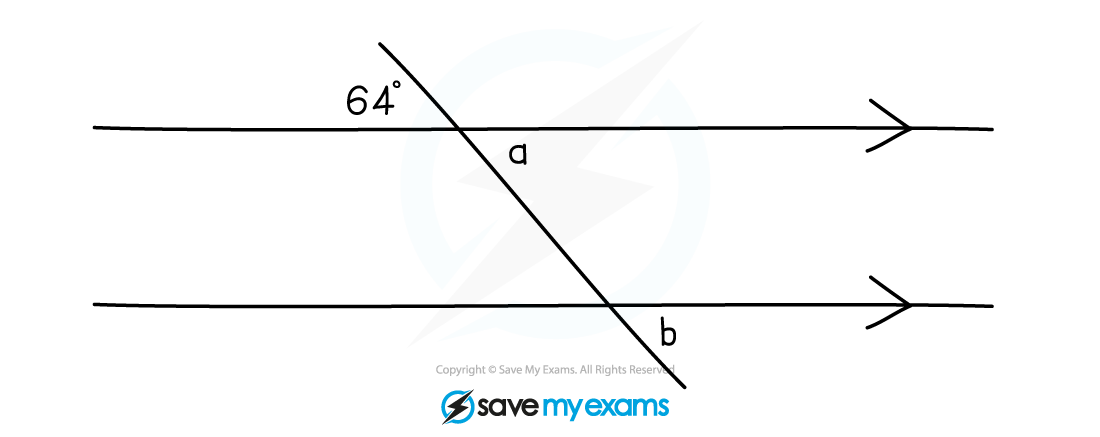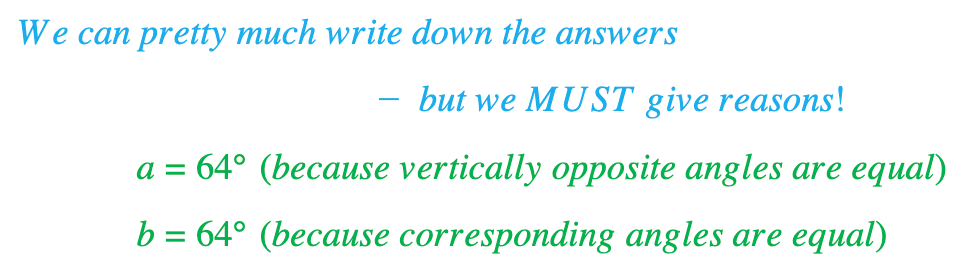# Edexcel IGCSE Maths 复习笔记 4.1.1 Angles in Parallel Lines

Edexcel IGCSE Maths 复习笔记 4.1.1 Angles in Parallel Lines

#### What are parallel lines?

• Parallel lines are lines that are always equidistant (ie the same distance apart) – no matter how far the lines are extended in either direction, they will never meet.

#### Working with angles in parallel lines

• There are 3 main rules:

1. Corresponding angles are equal

• A line cutting across two parallel lines creates four pairs of equal corresponding angles, as in the diagram below: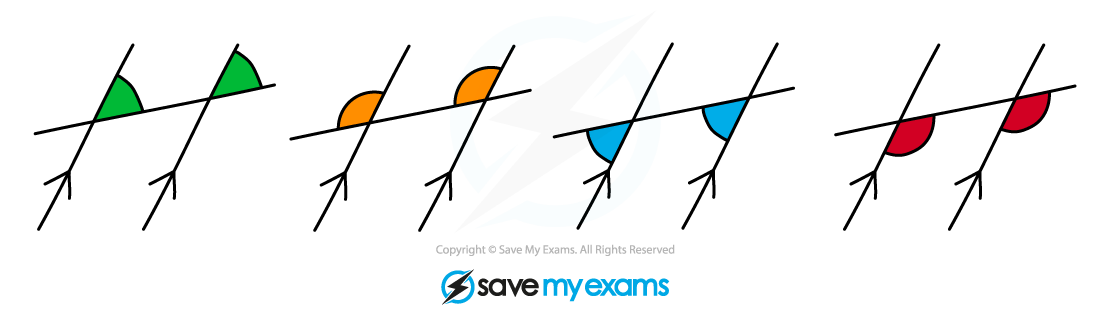•  Note: You may also have heard these referred to as ‘F angles’ – do not use that term in an exam or you will lose marks!

2. Alternate angles are equal

• A line cutting across two parallel lines creates two pairs of equal alternate angles, as in the diagram below: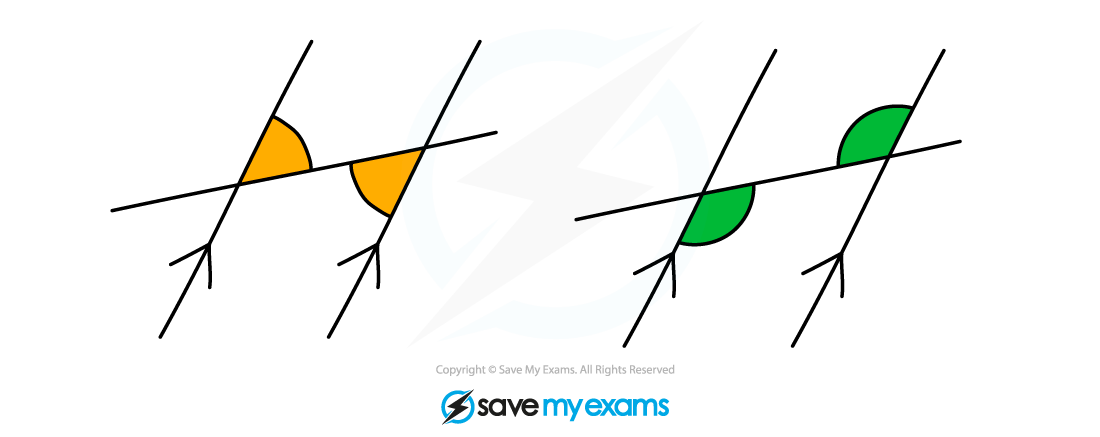• Note: You may also have heard these referred to as ‘Z angles’ – do not use that term on an exam or you will lose marks!

3. Co-interior angles add to 180°

• A line cutting across two parallel lines creates two pairs of co-interior angles
• In the diagram below, the two coloured angles on the left add up to 180°, as do the two coloured angles on the right:• Note: These are sometimes referred to as allied angles, which is fine. You may also have heard these referred to as ‘C angles’ – do not use that term on an exam or you will lose marks!

4. Vertically opposite angles are equal

• Whenever two straight lines cross, they create two pairs of equal vertically opposite angles, as in the diagram below:• Don’t forget this rule when answering parallel line questions! For example, in the following diagram the highlighted angles are equal: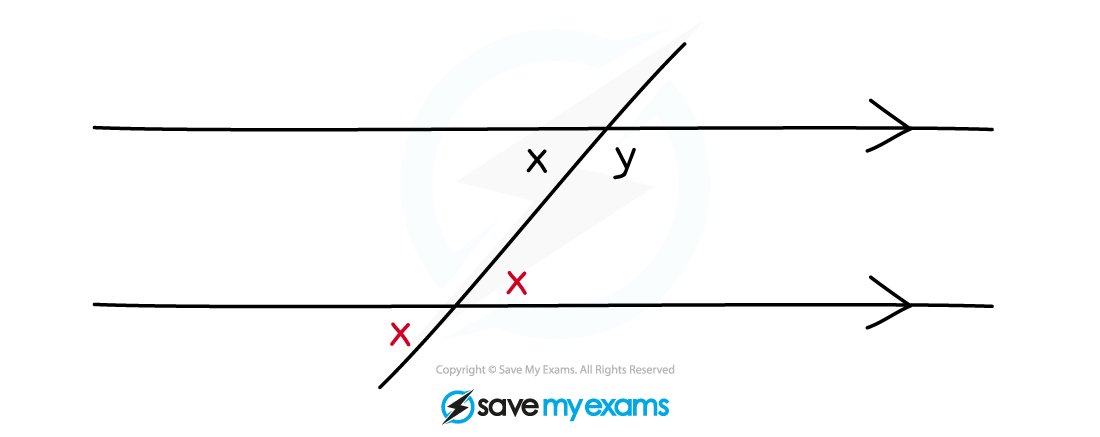• Note: vertically opposite angles are sometimes simply called opposite angles. Either term will get you the marks

5. Angles on a line add to 180°

• This rule is also still true with parallel line questions! In the following diagram, for example, the highlighted angles add up to 180°:Then:6. Just angle chase

#### Exam Tip

Do not forget to give reasons for each step of your working in an angles question.These are often needed to get full marks!

#### Worked Example